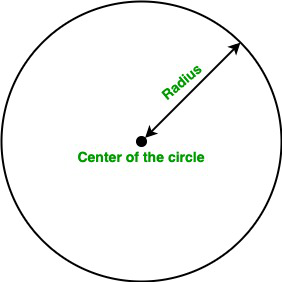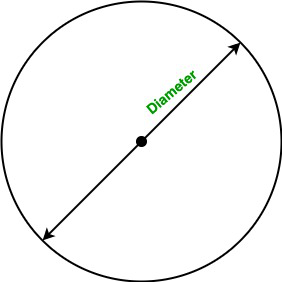# Java Program to Find the Perimeter of a Circle

• Difficulty Level : Basic
• Last Updated : 22 Jun, 2022

A circle is a simple shape consisting of all the points in the plane that are equidistant from a point known as the center of the circle. In this article, we will learn how to find the area of the circle.

Terminology:

• Perimeter: A quantity of measurement or boundary of the circle. It is basically the distance around a closed figure.
• Radius: The line segment from the center to any point of the circle is known as radius.
• Diameter: The line segment whose endpoints lie on the circle and passed through the center is known as the diameter of the circle. It is also known as the largest distance between any two points on the circle.

Perimeter Of A Circle:

• Using the radius of the circle:Formula:

Perimeter of the circle:  P =2 *  π * r

Here, r is the radius of the circle.

• Using the diameter of the circle:Formula:

Perimeter of the circle:  A =  π * d

Note: The value of PI in java is 3.141592653589793.

Example 1:

## Java

 `// Java program to find``// the perimeter of the circle``// using radius` `import` `java.io.*;` `class` `GFG {` `    ``static` `final` `double` `PI = Math.PI;` `    ``// Function to calculate the``    ``// perimeter of the circle``    ``static` `double` `Perimeter(``double` `r)``    ``{``      ``return` `2` `* PI * r;``    ``}` `    ``// Driver code``    ``public` `static` `void` `main(String[] args)``    ``{` `        ``// Radius``        ``double` `r = ``5``;` `        ``// Calling Perimeter function``        ``System.out.println(``"Perimeter of the circle is :"``                           ``+ Perimeter(r));``    ``}``}`

Output

`Perimeter of the circle is :31.41592653589793`

Time complexity: O(1)

Auxiliary space: O(1)

Example 2:

## Java

 `// Java program to find``// the area of the circle``// Using Diameter` `import` `java.io.*;` `class` `GFG {` `    ``static` `final` `double` `PI = Math.PI;` `    ``// Function to calculate the``    ``// perimeter of the circle``    ``// using Diameter``    ``static` `double` `Perimeter(``double` `D)``    ``{``        ``return` `PI * D;``    ``}` `    ``// Driver code``    ``public` `static` `void` `main(String[] args)``    ``{` `        ``// Diameter``        ``double` `D = ``20``;` `        ``// Calling Perimeter function``        ``System.out.println(``"Perimeter of the circle is :"``                           ``+ Perimeter(D));``    ``}``}`

Output

`Perimeter of the circle is :62.83185307179586`

Time complexity: O(1)

Auxiliary space: O(1)

My Personal Notes arrow_drop_up